# Algorithmic Trading in the Iowa Electronic Markets¶

## Abstract¶

The Iowa Electronic Markets are small, real-money financial markets designed to aggregate information about future events. The market microstructure of these markets is studied and a market making model is developed to provide liquidity for one set of securities offered by this exchange. A computer program was created to employ the market making model and profit from the market's inefficiencies. Using invested capital, the system traded 34% of the total market volume and achieved a Sharpe ratio of 9.9. This paper reveals the details of how this algorithmic trader worked to show how it functioned and the value it added to the Iowa Electronic Markets.

## Mechanics of the Iowa Electronic Market¶

• The 2008 Winner Takes All Presidential Election financial market had two securities:
• representing the Democratic candidate (DEM), Barack Obama
• representing the Republican candidate (REP), John McCain
• There were no explicit transaction costs from trading these securities in this market
• At maturity, each security pays \$1 if the security's candidate wins and \$0 if the candidate loses
• For these securities, a win is when the candidate captures more votes than his opponent as reported by The New York Times three days after the election and is not based on the electoral college

## Two Securities and One Asset¶

• Since there can be only one winner, the total payout of both securities at maturity is guaranteed to be equal to \$1. • To avoid arbitrage, the value of the two securities together must also be equal to \$1 for all times before maturity, ignoring any discount functions
• Price movements must be inversely correlated because when the value of one security goes up, the other must go down

• Although there are two securities, this market has only one risk factor and one real asset
• Buying one security is the same as selling the other
• securities = one of the two securities representing the two candidates

• asset or market asset = the one true asset that is is traded in this market

A long position in the market asset is defined as a long position in the Democratic candidate and a short position in the Republican candidate, and a short position in the market asset is the opposite.

## Market Information¶

• Three pieces of price information for each security; the bid price, the ask price, and the last trade price
• Minimum tick size for all prices is one tenth of a penny
• The last trade size, bid size, and ask size are not disclosed

Since there are two securities that are by definition inversely correlated, it simplifies things to invert the price of one of them so it is comparable to the other. For example, if these are the market prices:

In :
DEM_REP([(0.572, 0.602), (0.424, 0.45)], xsteps=(0.3, 0.7, 0.05))

Out:
BID ASK
DEM 0.572 0.602
REP 0.424 0.450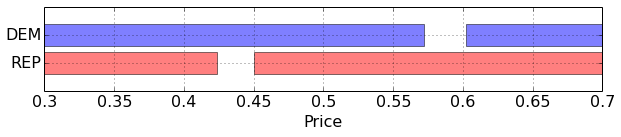Subtracting the REP bid-ask prices from 1 yields bid-ask prices for REPinv:

In :
DEM_REPinv([(0.572, 0.602), (0.424, 0.45)], xsteps=(0.5, 0.7, 0.05))

Out:
BID ASK
DEM 0.572 0.602
REPinv 0.550 0.576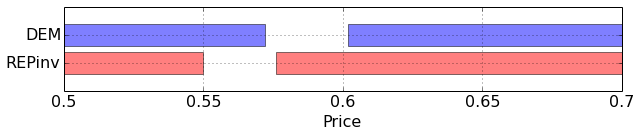REPinv has the same market exposure as DEM.

Notice that the REP bid price determines the REPinv ask price, and the REP ask price determines the REPinv bid price.

• The IEM allows market participants to buy and sell bundles of securities with the exchange itself for a fair price of \$1 • A bundle is the pair of securities DEM and REP • This allows a trader two alternatives to trade into a position: • buy the one security they want • buy bundles and then sell the other undesired security ## What is the Market Asset's true bid and ask prices?¶ • bid price is the maximum of the DEM and REPinv bid prices • offer price is the minimum of the DEM and REPinv offer prices If a trader wanted to trade in this market it would be efficient and rational for them to only trade using the asset's true bid and ask prices. These two prices make up what can be considered to be the good spread and the other two prices make up the bad spread. In : Good_Bad([(0.572, 0.602), (0.424, 0.45)], xsteps=(0.5, 0.7, 0.05))  Out: BID ASK Good spread 0.572 0.576 Bad spread 0.550 0.602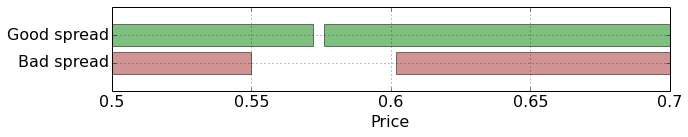#### My view of the market asset's 'Fair Value' is the midquote of the Good Spread¶ This pricing methodology does not allow arbitrage, but when the good spread is crossed (the bid is greater than the ask), the fair price is undefined. ## Market Efficiency¶ • It would be inefficient for a trader to use a market order to buy the market asset on the bad offer or sell the market asset at the bad bid • Doing so would be considered a price-taking violation of individual rationality, as discussed in Oliven and Rietz (2004) • The researchers found that in the 1992 Presidential election, 37.7% of market orders made this error # Market Activity¶ Plots of Market Activity during the time of this research project. In : activity_plot(["Mid Price"], ["r"])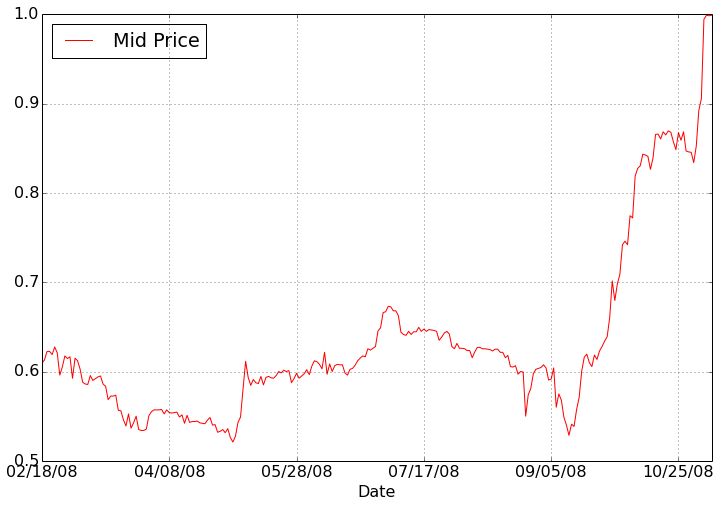In : activity_plot(["Volatility"], ["r"])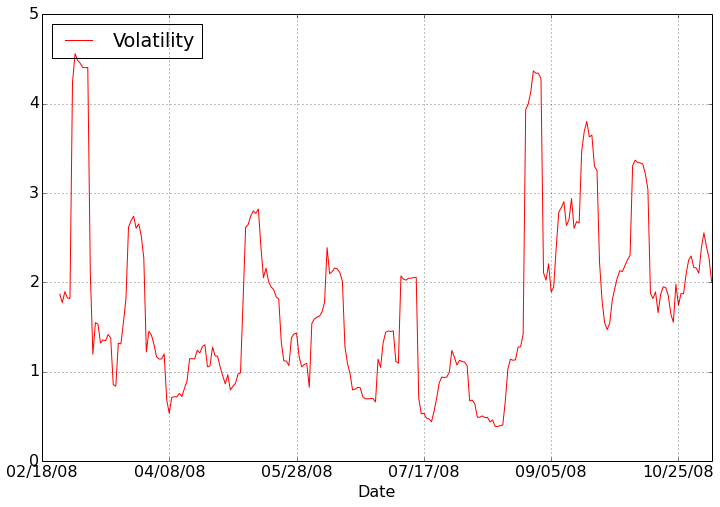In : activity_plot(["Good Spread", "Bad Spread"], ["green", "brown"])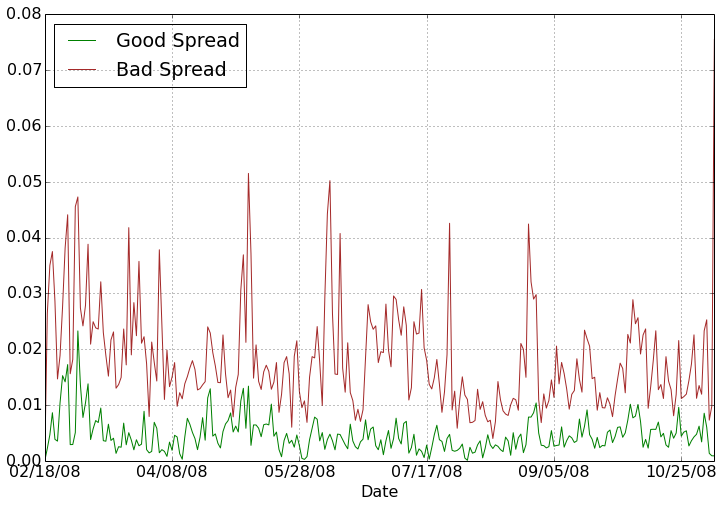In : activity_plot(["Total Market Value", "My$ Traded"], ["k", "r"])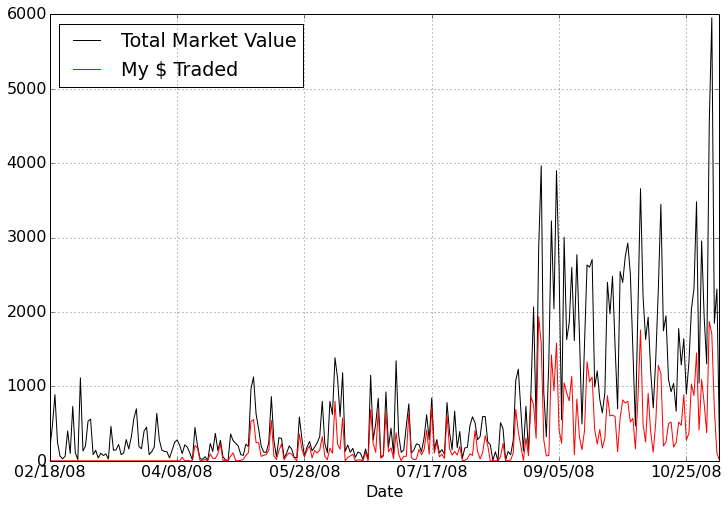# Arbitrage¶

• In this market it is possible for arbitrage opportunities to arise
• Oliven and Rietz (2004): market-making violation of individual rationality
• A trader adds a limit order to one of the order books that causes the good spread to become crossed
• A crossed market is when the bid price exceeds the ask price

For example, in this market:

In :
DEM_REP([(0.572, 0.602), (0.424, 0.45)], xsteps=(0.3, 0.7, 0.05))

Out:
BID ASK
DEM 0.572 0.602
REP 0.424 0.450In :
DEM_REPinv([(0.572, 0.602), (0.424, 0.45)], xsteps=(0.3, 0.7, 0.05))

Out:
BID ASK
DEM 0.572 0.602
REPinv 0.550 0.576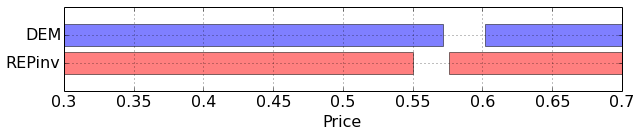A DEM bid of 0.580 would have this result:

In :
DEM_REPinv([(0.58, 0.602), (0.424, 0.45)], xsteps=(0.3, 0.7, 0.05))

Out:
BID ASK
DEM 0.58 0.602
REPinv 0.55 0.576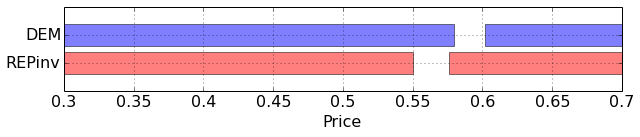In :
Good_Bad([(0.58, 0.602), (0.424, 0.45)], xsteps=(0.3, 0.7, 0.05))

Out:
BID ASK
Good spread (crossed) 0.58 0.576
Bad 0.55 0.602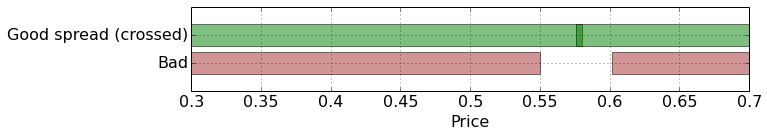The arbitrage opportunity is to sell both the DEM and REP securities at their respective bid prices and then buy bundles by trading with the exchange.

In :
DEM_REP([(0.58, 0.602), (0.424, 0.45)], xsteps=(0.3, 0.7, 0.05))

Out:
BID ASK
DEM 0.580 0.602
REP 0.424 0.450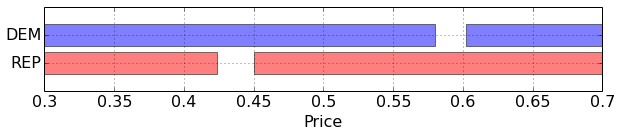This is profitable because the pair of securities can be sold at a price of 1.004 and bought back from the exchange with a bundled transaction for 1 dollar. The exchange has a special market order for buying and selling bundles using the sum of the displayed bid or ask prices that ensures equal numbers of each security are traded.

## Market Gaming¶

• The absence of displayed order sizes in this market creates the potential for traders to game this market or behave in a way that frustrated the system's ability to determine the efficient price of the market asset.
• A limit order for one share appears to other market participants appears similar to limit orders for larger sizes.
• Do traders place one share orders in an attempt to alter other market participants' perception of current prices?
• It is clear that traders placed one share orders with some frequency because sometimes those market participants would also inadvertently create an arbitrage opportunity.
• About 10% of the system's 1,267 arbitrage attempts resulted in a trade of only one bundle, indicating that the smaller order for the two securities was for one share.

#### Why would a trader do this?¶

• Is it a trader's attempt to draw more favorably priced orders to the opposite side of the order book they intend to trade?
• Are there politically motiviated traders attempting to alter the market price?

# Market Making Model¶

Consider an exponential utility function to evaluate changes in wealth, W:

$$U(W) = -e^{-\lambda W}, \lambda > 0$$

Assume that $W$ is distributed normally with a mean of $\mu$ and a variance of $\sigma^2$. The expectation of $U(W)$ is then given by:

$$\mathbf{E}U(W) = \frac{1}{\sigma \sqrt{2\pi}} \int_{-\infty}^{\infty} -e^{-\lambda W} e^{-\frac{(W-u)^2}{2\sigma^2}} dW$$

By re-arranging the terms and employing calculus, one can reduce this to:

$$\mathbf{E}U(W) = -e^{-\lambda (\mu - \frac{\lambda \sigma^2}{2})}$$

Maximizing the expected utility of changes in wealth $W$ is then equivalent to maximizing the expression

$$\mu - \frac{\lambda \sigma^2}{2}$$

The exponential utility function is equivalent to the mean variance utility function when changes in wealth are normally distributed.

We can then write the market making model's utility function as:

$$U(W) = \mathbf{E}[W] - \frac{\lambda}{2} \mathbf{Var}[W]$$

The market maker can estimate the expected increase in wealth when it buys a security on its bid price or sells at its ask price:

$$\mathbf{E}[W] = s \left | X \right | - G(Z)(X+A-D)$$

where:

• $Z$ is the total size of the counterparty's trade, which may be partly or completely filled by the market maker.
• $A$ is the market maker's current position before the trade
• $D$ is the desired position, making the difference $A-D$ the current undesired position.
• $X$ is the potential alteration to the market maker's portfolio, which will be positive (negative) when the market maker is buying (selling).
• $s$ is market maker's per-share compensation for altering its portfolio, aka the spread
• The total compensation $s \left | X \right |$ is positive when buying or selling.
• The function $G(Z)$ is a function modeling the permanent impact of the trade.

If:

• $P_{post}$ is the market asset's efficient price sufficiently far enough into the future after the trade that the temporary impact has completely decayed
• $P$ is the price immediately before the trade

Then:

function $G(Z)$ becomes an estimate of the price change $P_{post} - P$:

$$G(Z) = P_{post} - P$$

The function $G(Z)$ must be linear in $Z$ to enforce a no arbitrage condition on the market.

The linear market impact model is:

$$\frac{P_{post} - P}{P} = \gamma Z$$

Market impact is then a linear function of the constant $\gamma$.

Defining $Y=Z-X$ as the estimated size of the trade filled by other market participants at the same price, the market impact function $G(Z)$ becomes

$$G(Z) = \gamma P (X + Y)$$

The estimate of the expected profit from a buy or sell trade is:

$$\mathbf{E}[W] = s \left | X \right | - \gamma P (X + Y)(X+A-D)$$

The variance of the expected increase in wealth comes from the variance of changes in the undesired position value, including the new position $X$. The market making model can estimate the variance of net wealth as:

$$\mathbf{Var}[W] = (\sigma \sqrt{t} P(X+A-D))^2$$

where:

• $P$ is the efficient price of the market asset
• so the quantity $P(A-D+X)$ is the value of the new undesired position measured in dollars.
• notice that $X$ can increase or decrease the variance of the market maker's position, depending on the value of $A-D$.
• $\sigma$ is the estimated one-day volatility of the market asset
• $t$ is the expected time in days the market maker will hold the position before receiving an offsetting trade.
• volatility scales by the square root of time.

Substituting $\mathbf{E}[W]$ and $\mathbf{Var}[W]$ into the utility function $U(W) = \mathbf{E}[W] - \frac{\lambda}{2} \mathbf{Var}[W]$ yields:

$$\mathbf{U}(W) = sX - \gamma P (X + Y)(A-D+X) - \frac{\lambda}{2} (\sigma \sqrt{t} P(A-D+X))^2$$

To find the optimal number of shares the market maker would be willing to buy or sell at a price earning a spread of $s$, simply calculate the first derivative of $\mathbf{U}(W)$, set the result equal to zero, and solve for $X$.

The optimal number of shares for the market maker to be willing to buy on the bid or sell at the offer becomes:

$$X_{bid} = \max\Biggl[0, \frac{s - \gamma P (Y + A - D) - \lambda \sigma^2 t P^2 (A - D)}{2 \gamma P + \lambda \sigma^2 t P^2}\Biggr]\\ X_{ask} = \max\Biggl[0, \frac{s - \gamma P (Y - A + D) + \lambda \sigma^2 t P^2 (A - D)}{2 \gamma P + \lambda \sigma^2 t P^2}\Biggr]$$

Interactive Model to help visualize the model available here

# Trading Results¶

In :
activity_plot(["Total Profit", "MM Profit", "Arb Profit"], ["k", "r", "b"])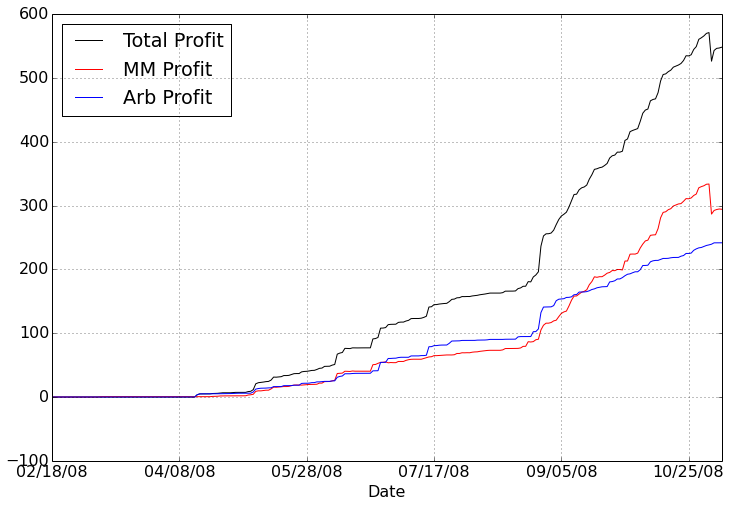In :
activity_plot(["MM Trades", "Arb Attempts"], ["k", "r"])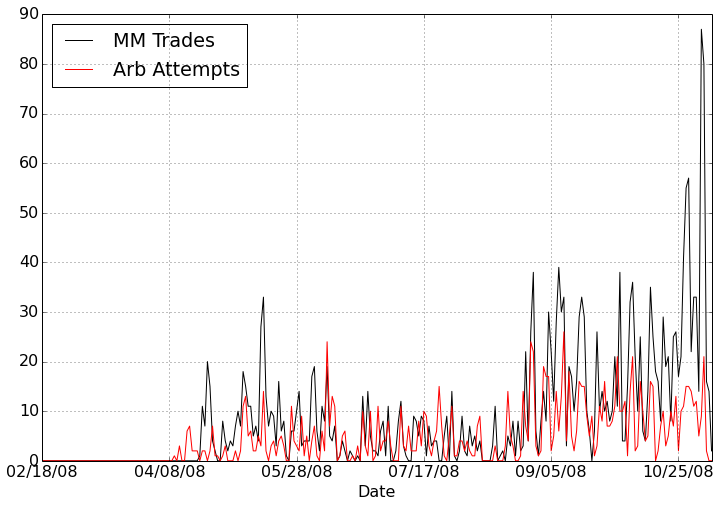### Decompose Market Making Profits into Positioning Profits and Spread Profits¶

• The spread profit is the profit the market making algorithm earns from buying at prices less than the mid price and selling at prices higher than the mid price. Spread profits accumulate with every market making trade.
• The positioning profit is the profit the algorithm earns from favorable price movements of its asset holdings. Positioning profits accumulate inbetween market making trades.

Which will be greater: spread profits or positioning profits?

In :
activity_plot(["MM Profit", "Spread Profit", "Positioning Profit"], ["r", "k--", "b--"])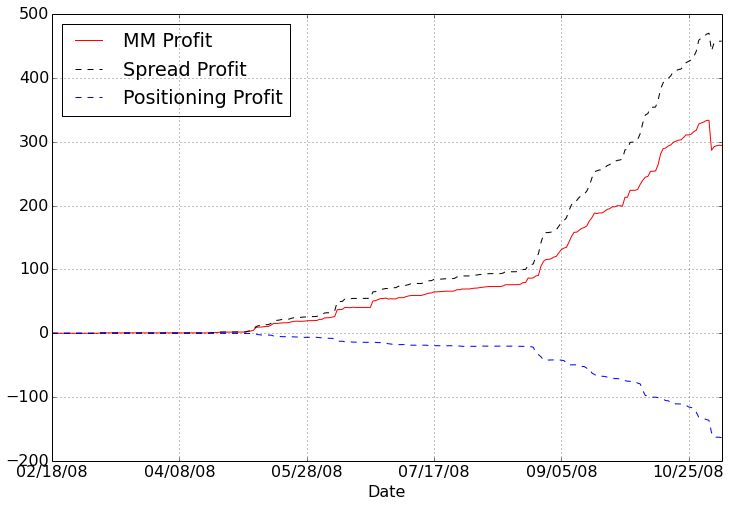# Discussion¶

• How does the structure of the Iowa Electronic Markets create trading opportunities?
• Compare/contrast with other markets
• How did I benefit this market and its goal of aggregating information?
• Did human traders benefit from my presence in this market? Was there any harm?
• Implementation details
• What about 2004, 2012, and 2014?